# Addition and Subtraction of Dissimilar Fractions – Fractions with Different Denominators

Okay. Here’s the thing. The addition or subtraction of dissimilar fractions (unlike fractions) is slightly different compared to the addition or subtraction of similar fractions (like fractions).

For the addition or subtraction of similar fractions, we are dealing only with the numerators. If you missed my previous lesson about it, you can review my previous discussion about the addition and subtraction of similar fractions.

Meanwhile, for the addition or subtraction of dissimilar fractions, it means that we will be dealing both with numerators and denominators.For you to proceed with this lesson easily, please make sure that you have the full understanding of the following prerequisite topics:

1. Addition and subtraction of similar fractions (or like fractions).

2. Finding equivalent fractions.

3. Finding the “Least Common Denominator” or LCD.

### How to Add Unlike Fractions (dissimilar fractions) Step-by-step.

To make it easy, I divided the procedure on how to add or subtract unlike fractions into two (2) basic steps as follows:Step 1. Change all unlike fractions (dissimilar fractions) into like fractions (similar fractions).

In order to this, we need to change the denominators of the fractions into a common denominator. Remember that similar fractions has the same or common or like denominators.

The common denominator must be a multiple of all different denominators. Meaning to say, the common denominator can be divided by all the denominators without any remainder.

There are infinite numbers of common denominator for any fractions, but we will consider only the smallest or the lowest one. This is what do we called the least common denominator or LCD.

Finally, obtain the equivalent fractions of those fractions using the common denominator obtained.Step 2. Proceed with the same procedure as adding or subtracting similar fractions.

We will follow the same procedure of adding or subtracting similar fractions as follows:

• Add or subtract the numerators.
• Copy the denominators.
• Convert the final answer in the lowest term.

Let us have an example.

### Adding Unlike Fractions – Examples

Example No.1 Find the sum of 1/4 and 1/2.First step is to find the least common denominator of 1/4 and 1/2. It is the same as finding the least common multiple of 4 and 2.

Our least common denominator is 4 as shown above. The next to do next is to put 4 as our new denominators.

Then, we will find the equivalent fractions of our original fractions with 4 as the denominator.

1/4 will not change. 4 is already the denominator.

The equivalent fraction of 1/2 with 4 as the denominator is 2/4.

Now, we have 1/4 plus 2/4. We can easily add this now because they are similar fractions.

1/4 plus 2/4 is equal to 3/4. We just simply add the numerators.

Since our sum which is equal to 3/4 is already in the lowest term, 3/4 is the final answer.

Example No.2 Find the sum of 2/3 and 1/4.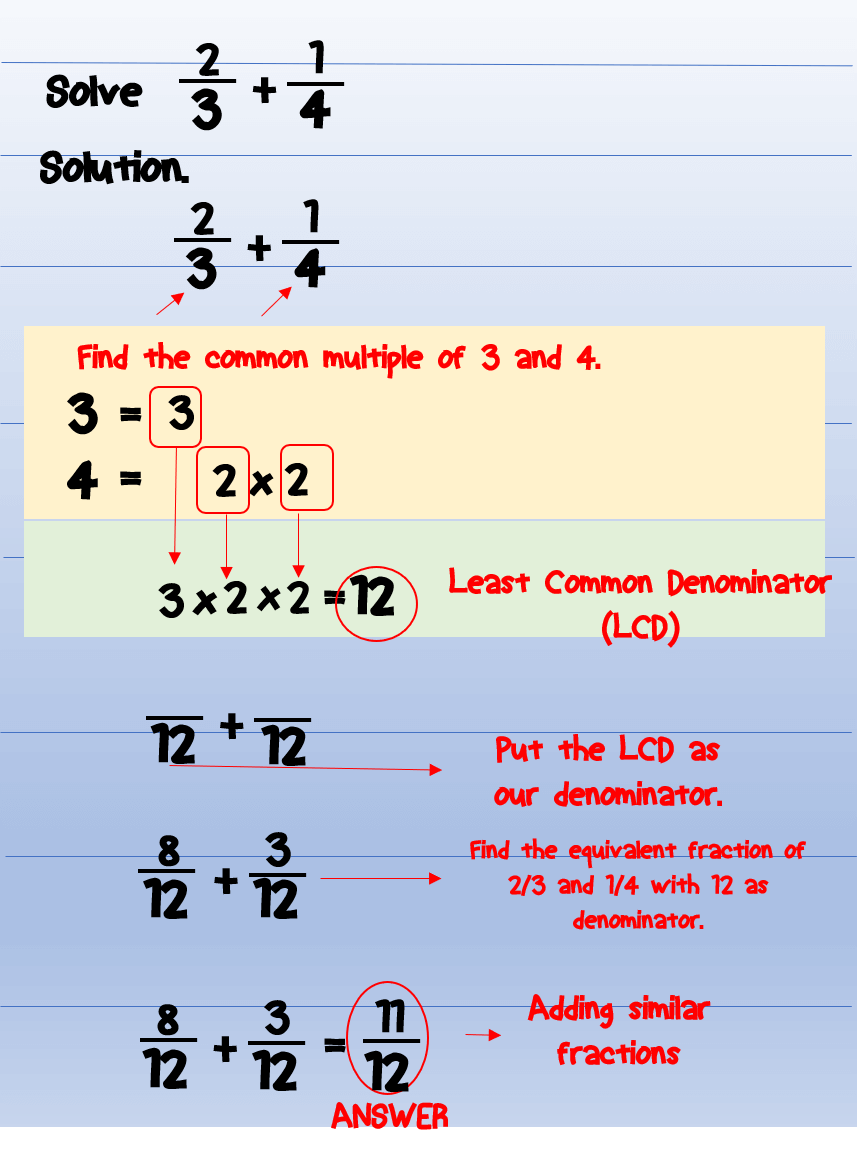The first thing that we want to do is to change those fractions into similar fractions.

In order to do that, we must find the LCD. The LCD is the least common multiple of 3 and 4.

In our solving above, the least common multiple of 3 and 4 is 12. We will use 12 as our new denominator.

The next thing to do is to find the equivalent fraction of 2/3 and 1/4 with 12 as the denominator.

The equivalent fraction of 2/3 with 12 as the denominator is 8/12.

The equivalent fraction of 1/4 with 12 as the denominator is 3/12.

Since we have already changed them into similar fractions, we can proceed with adding their numerators.

8/12 plus 3/12 is equal to 11/12.

More example of adding unlike fractions.

Example No.3 Find the sum of 1/6 and 3/4.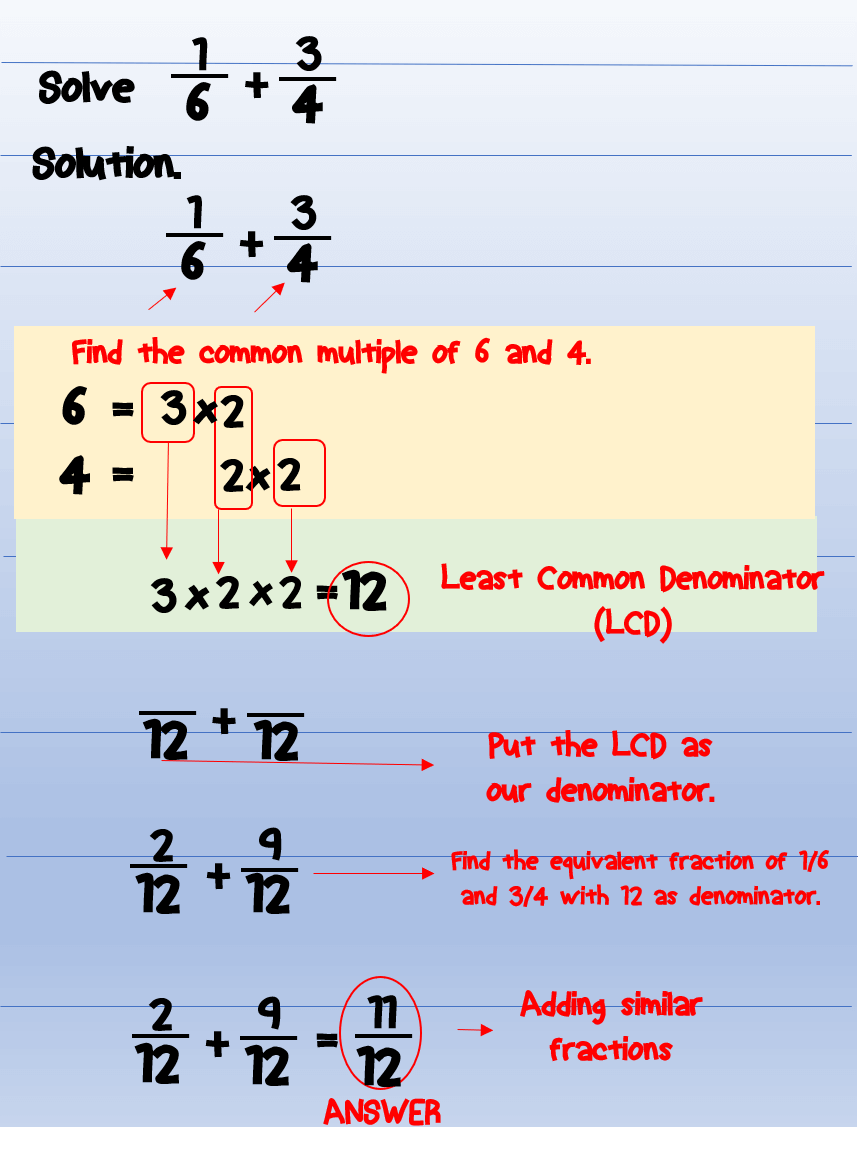Example No.4 Find the sum of 4/5 and 3/4.Example No.5 Find the sum of 1/6, 2/9, and 5/18.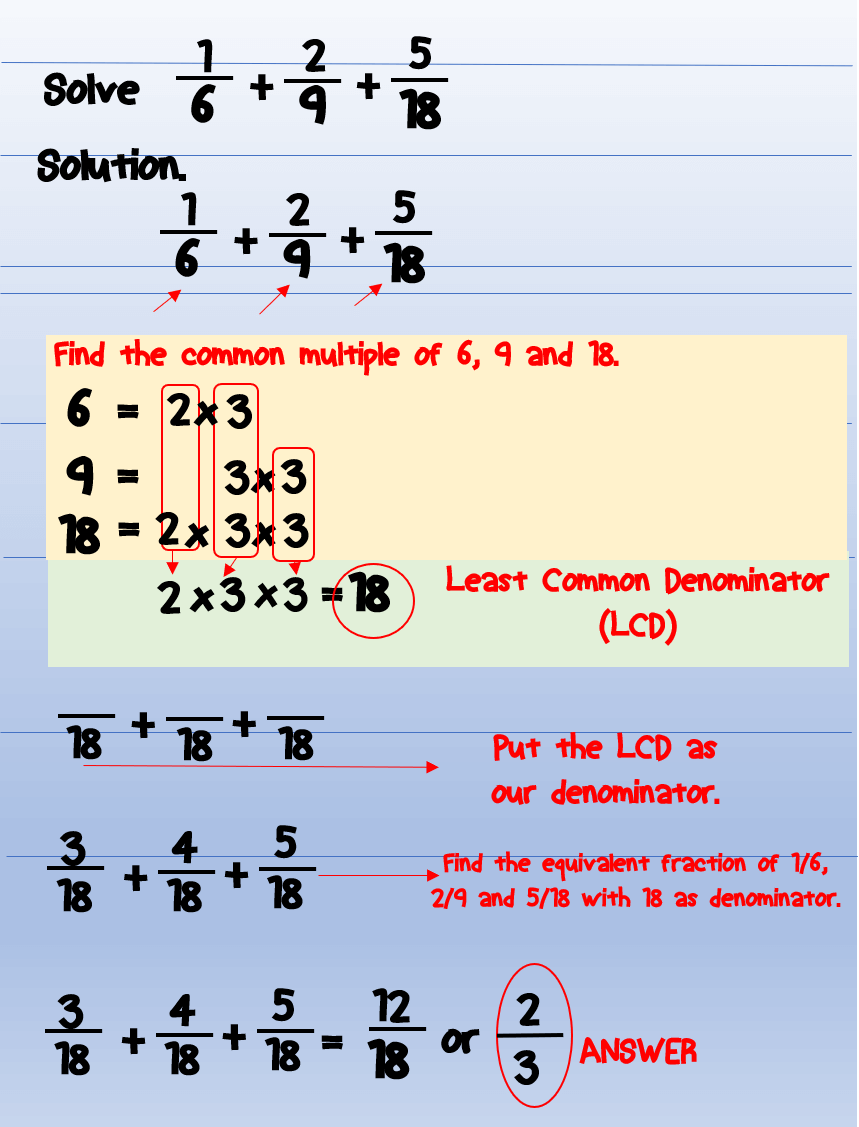### How to Subtract Unlike Fractions (dissimilar fractions) – Steps

First, we will change dissimilar fractions into similar fractions by finding the least common denominator.

Second, add them like similar fractions.

And then finally, convert the answer in the lowest term.

### Subtracting Unlike Fractions – Examples

Example No.6 Subtract 3/4 from 4/5.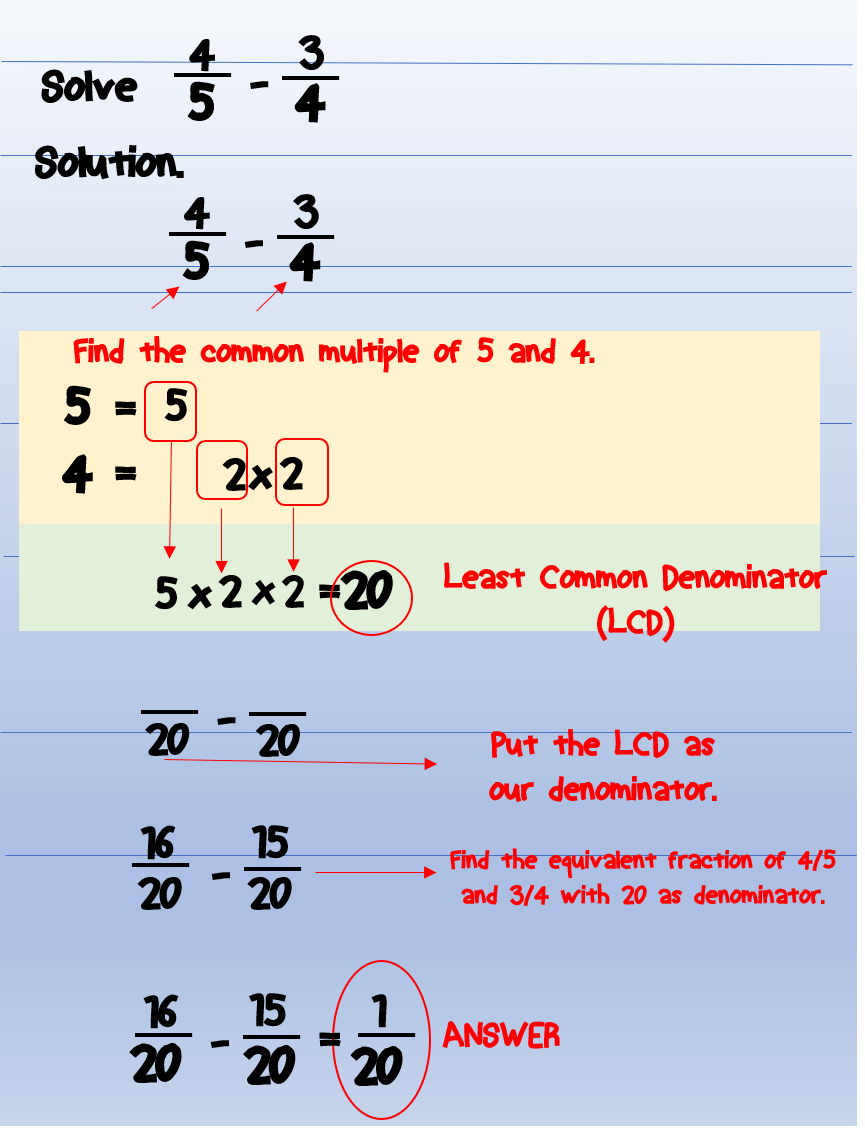What will be our least common denominator? The least common multiple of 5 and 4 is 20. Thus, 20 is our least common denominator.

The equivalent fraction of 4/5 with 20 as the denominator is 16/20.

The equivalent fraction of 3/4 with 20 as the denominator is 15/20.

Since we already have similar fractions, perform the subtraction.

16/20 minus 15/20 is equal to 1/20.

1/20 is in the lowest term.

Thus, 1/20 is the final answer.

Example No.7 Subtract 1/4 from 2/3.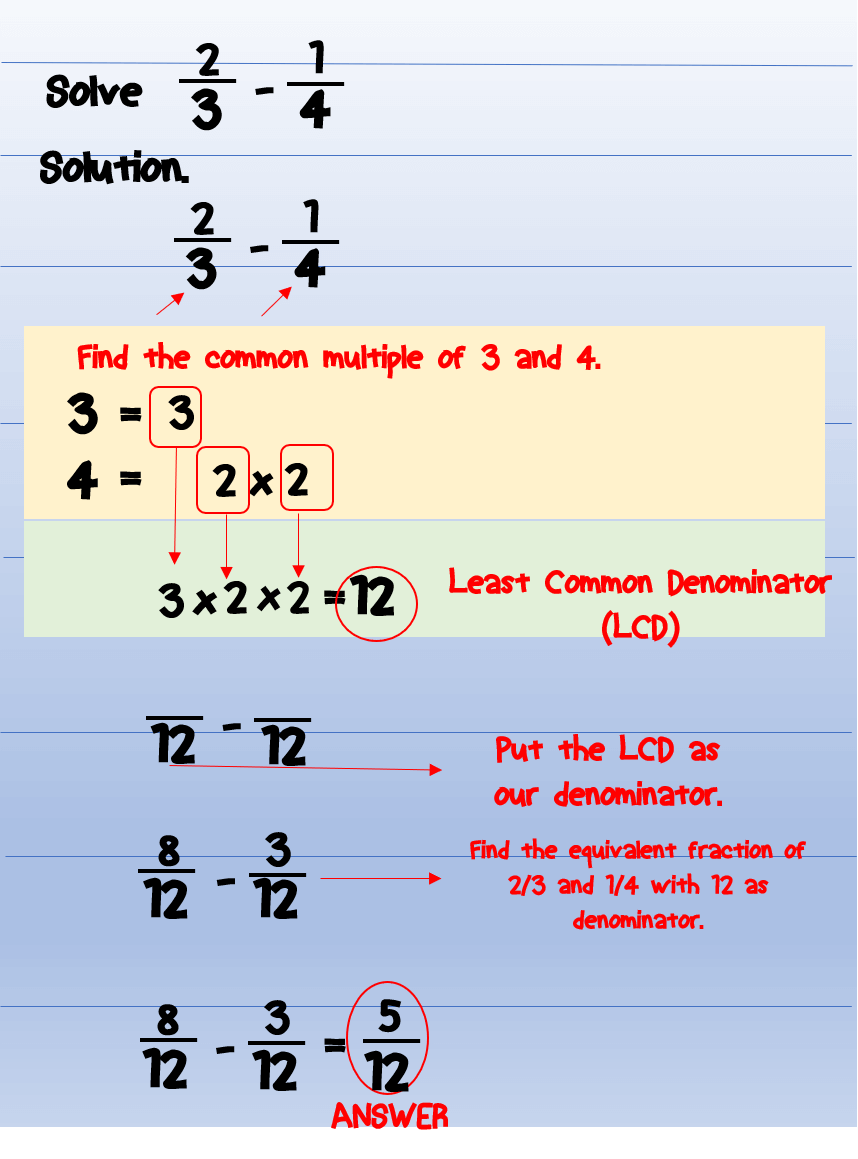First, solve for the least common denominator. The least common denominator is 12 as shown in our calculation.

The equivalent fraction of 2/3 with 12 as the denominator is 8/12.

While the equivalent fraction of 1/4 with 12 as the denominator is 3/12.

Thus, 8/12 minus 3/12 is equal to 5/12.

5/12 is already in the lowest term.

Thus, 1/20 is the final answer.

### Summary – Adding and Subtracting Unlike Fractions

1. To add or subtract dissimilar fractions (or unlike fractions), change dissimilar fractions into similar fractions (or like fractions). Then, add or subtract the fractions as similar fractions. The sum or difference must in the lowest term.
2. To change dissimilar fractions (or unlike fractions) into similar fractions (or like fractions0, we need to find the Least Common Denominator or LCD.

### Related Topics

If you have some questions or problems related to the above topic, please feel free to drop it in the comments section below.

Enjoy!

1.Chyke says:

I like the way you patiently explained every step that it is easily understandable to a child. I think parents will love this site. I am just seeing it for the first time. As a child, I wasn’t too good at math but then we didn’t have access to materials like this and patient teachers such as you. Or maybe we did have good teachers but were too resigned to follow what they taught.

1.Faisal says:

Thank you Chyke. I really appreciate it. I love teaching kids and I love sharing my math knowledge to them.

2.JB Symonette says:

Your explanation was very organized and systematic. Honestly, I was never much of a math wiz, but your use of pictures was very effective. The steps were also extremely clear. Great job!

1.Faisal says:

Thank you. I love teaching. Please come back for more my interesting posts.

3.A Phill says:

As a father , I am teaching my daughter simple and basic maths and seeing your website and how you have painstakingly analysed every step that it is easily understandable to a child.
I am bookmarking your blog because I can learn more and transfer the skills to my girls. I use Kumon to teach them at the moment but when it comes to the logic I need to delve deeper just like you have done.
Growing up I loved maths and further maths but now into construction but not lost my love for the subject.

Thanks

1.Faisal says:

Exactly. This is actually one my purpose – to help parents or kids to understand basic math topic better.

Regards.

4.Jukka says:

This was actually a really well thought out lesson, thank you for that! I was always decent with math in school so this was nothing new really but I can’t actually remember that we would have learned to find the LCD the way you showed here. It’s really simple and logical and I actually learned something new :).

1.Faisal says:

You’re welcome. I tried to present all the lessons in its most basic approach.

5.Anne Santiago says:

This post contains a very detailed explanation in adding and subtracting fractions. It present an effective method on finding the LCD. I could say this is very helpful to pupils who just started learning fractions. I also want to share my idea on dealing with fractions but I think this is suitable for higher grade levels.

To add and subtract fractions successfully is to make the rules stick to your memory.

Rules are:
Same denominator:
Add both numerators then reduce. The result would be the final answer.
Different denominator (4 steps):
1. Multiply the numerator of first fraction to the denominator of second fraction. The result is the new numerator of first fraction.
2. Multiply the numerator of the second fraction to the denominator of first fraction. The result is the new numerator of second fraction.
3. Multiply both denominators. The result is the common denominator for two fractions.

To make it stick to your memory:
Rules for subtraction:
Same denominator:
Subtract second numerator from first then reduce. The result would be the final answer.
Different denominator (4 steps):
1. Multiply the numerator of first fraction to the denominator of second fraction. The result is the new numerator of first fraction.
2. Multiply the numerator of the second fraction to the denominator of first fraction. The result is the new numerator of second fraction.
3. Multiply both denominators. The result is the common denominator for two fractions.
4. Subtract new second numerator from first new numerator. The result is now the answer.

To make it stick to your memory:
Same numerator: